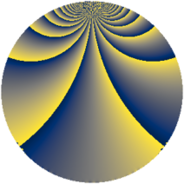Properties

 Label 2880.1.cdLevel $2880$ Weight $1$ Character orbit 2880.cd Rep. character $\chi_{2880}(1409,\cdot)$ Character field $\Q(\zeta_{6})$ Dimension $8$ Newform subspaces $1$ Sturm bound $576$ Trace bound $0$

Related objects

Defining parameters

 Level: $$N$$ $$=$$ $$2880 = 2^{6} \cdot 3^{2} \cdot 5$$ Weight: $$k$$ $$=$$ $$1$$ Character orbit: $$[\chi]$$ $$=$$ 2880.cd (of order $$6$$ and degree $$2$$) Character conductor: $$\operatorname{cond}(\chi)$$ $$=$$ $$45$$ Character field: $$\Q(\zeta_{6})$$ Newform subspaces: $$1$$ Sturm bound: $$576$$ Trace bound: $$0$$

Dimensions

The following table gives the dimensions of various subspaces of $$M_{1}(2880, [\chi])$$.

Total New Old
Modular forms 72 16 56
Cusp forms 24 8 16
Eisenstein series 48 8 40

The following table gives the dimensions of subspaces with specified projective image type.

$$D_n$$ $$A_4$$ $$S_4$$ $$A_5$$
Dimension 8 0 0 0

Trace form

 $$8 q + O(q^{10})$$ $$8 q + 4 q^{21} + 4 q^{25} + 12 q^{29} + 8 q^{45} + 4 q^{49} - 4 q^{69} + 4 q^{81} + O(q^{100})$$

Decomposition of $$S_{1}^{\mathrm{new}}(2880, [\chi])$$ into newform subspaces

Label Dim. $$A$$ Field Image CM RM Traces $q$-expansion
$a_{2}$ $a_{3}$ $a_{5}$ $a_{7}$
2880.1.cd.a $8$ $1.437$ $$\Q(\zeta_{24})$$ $D_{12}$ $$\Q(\sqrt{-5})$$ None $$0$$ $$0$$ $$0$$ $$0$$ $$q-\zeta_{24}^{11}q^{3}+\zeta_{24}^{2}q^{5}+(-\zeta_{24}^{3}+\cdots)q^{7}+\cdots$$

Decomposition of $$S_{1}^{\mathrm{old}}(2880, [\chi])$$ into lower level spaces

$$S_{1}^{\mathrm{old}}(2880, [\chi]) \cong$$ $$S_{1}^{\mathrm{new}}(1440, [\chi])$$$$^{\oplus 2}$$